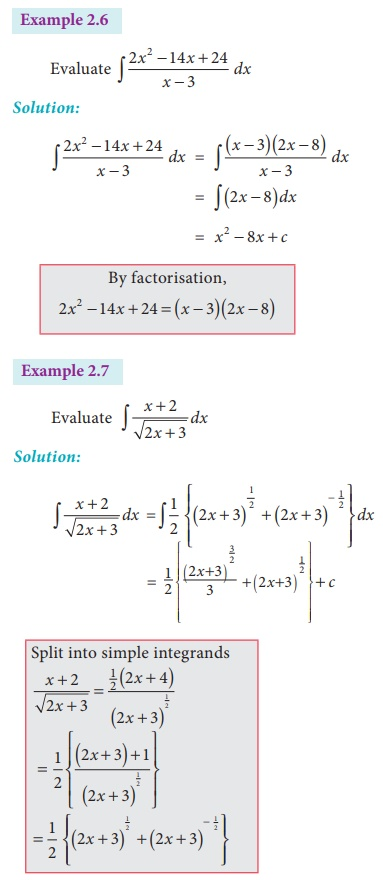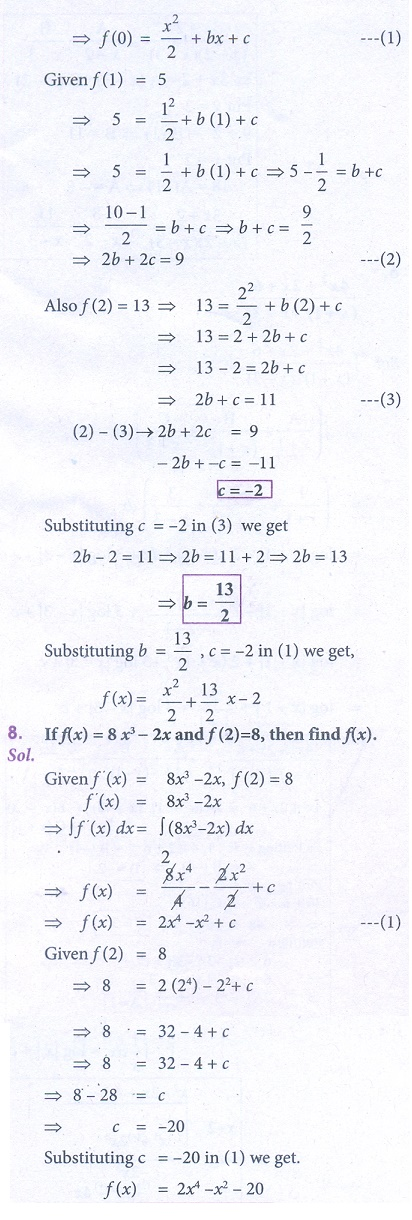Home | | Business Maths 12th Std | Integration by decomposition: Type I

# Integration by decomposition: Type I

The integrals which are directly obtained from their corresponding derivatives are known as the standard results of integration.

Integration by decomposition

Apply this method, whenever the integrand is impossible to integrate directly, but it can be integrated after decomposing into a sum or difference of integrands whose individual integrals are already known.

Note

The integrals which are directly obtained from their corresponding derivatives are known as the standard results of integration.

## Some standard results of integration

Type: IExercise 2.1

Integrate the following with respect to x.7. If f ′(x) = x + b, f (1) = 5 and f (2) = 13, then find (x)

8. If f ′(x) = 8x3 − 2x and f (2) = 8, then find f (x)